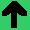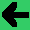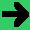# Lab 5: Modify Space Wars Physics

1. Turn off the gravity of the sun (2 points).
2. Reimplement the gravity from the sun using good old physics (3 points).
1. I did it by first getting it moving in the Ship.cs class (acceleration += (0,1,0))
2. Then I calculate gravity (in that same direction) first with a constant distance (200).
3. Then with a distance based on distance from sun (I guess making each pixel a metre). I made the sunWeight 4.0e+16;
4. Then I figured out the direction vector from the sun and applied it to that.
3. Put in weights between the two ships and have them act as the sole attractors of each other. I found it difficult to get the other ships location and used
SpacewarScreen tempScreen = (SpacewarScreen)SpacewarGame.currentSreen;
and had to relax some protections from private to public (2 points).
4. Combine the forces so the sun and the objects attract. (I didn't do this.) (2 points)
5. Make the sun move; I didn't do this, and it may be tricky. (1 point)